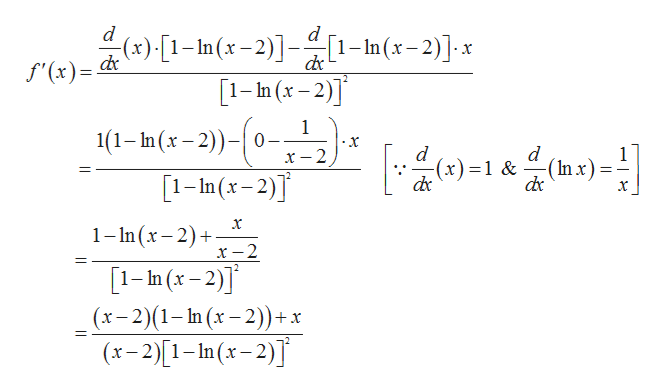# Differentiate f and find the domain of f. (Enter the domain in interval notation.)f(x) = x/1-ln(x-2)

Question
19 views

Differentiate f and find the domain of f. (Enter the domain in interval notation.)

f(x) = x/1-ln(x-2)
check_circle

Step 1

Given,

Step 2

Differentiating with res...help_outlineImage Transcriptionclosed (x) 1-In(x-2)] [1-In(x-2)]x f'(x) [1-In (x-2)] 1 1(1- h(x-2))-0- .X d x-2 (x)1 &(x) [1-In(x-2) х 1-n (x-2) _ x-2 1-In (x-2)] (x-2)(1-In (x-2))+x (x-2)1-In (x-2)] _ fullscreen

### Want to see the full answer?

See Solution

#### Want to see this answer and more?

Solutions are written by subject experts who are available 24/7. Questions are typically answered within 1 hour.*

See Solution
*Response times may vary by subject and question.
Tagged in

### Calculus